Anzeige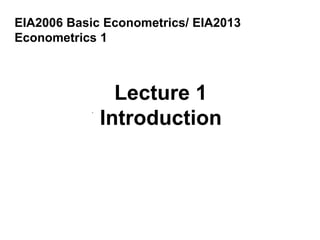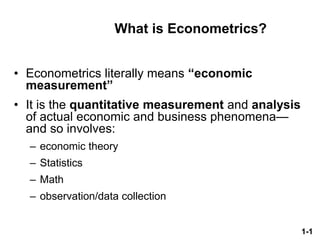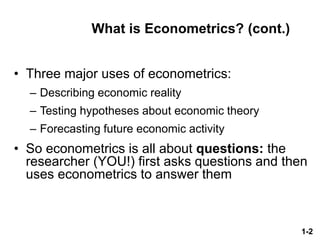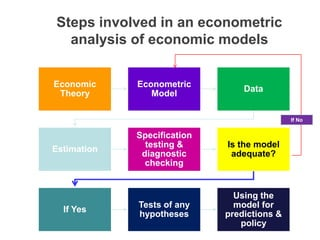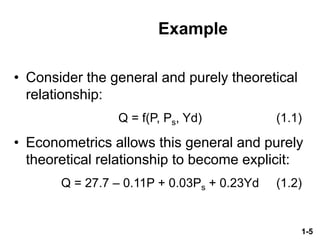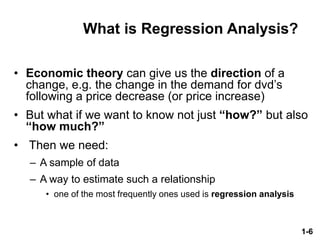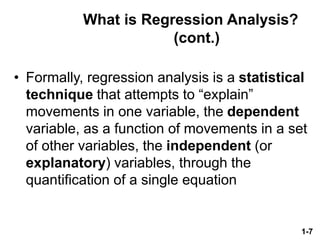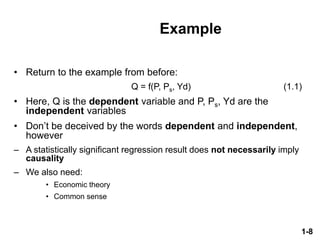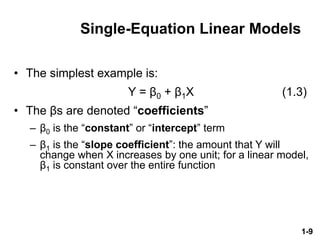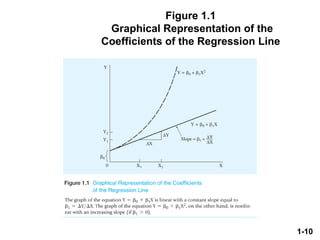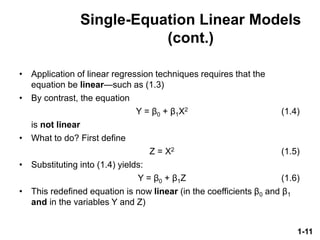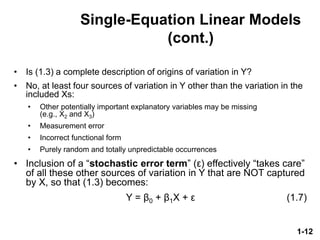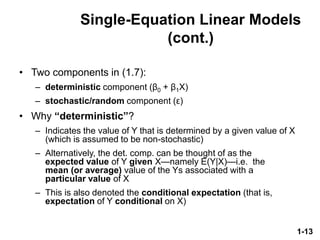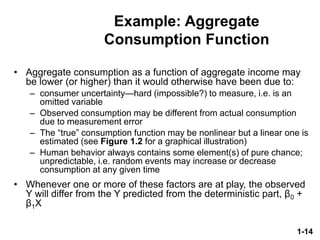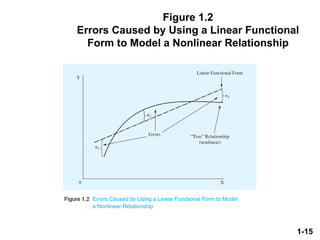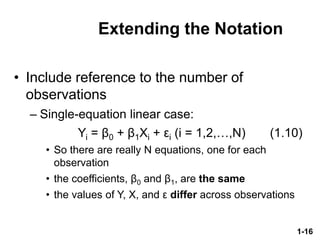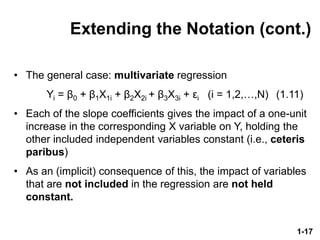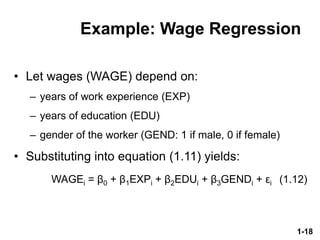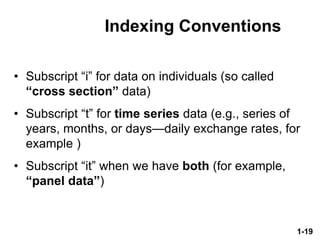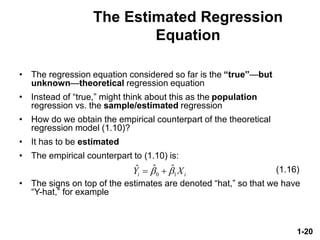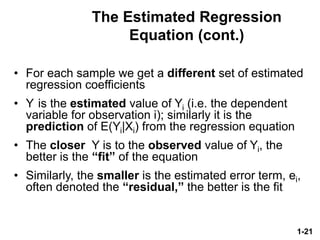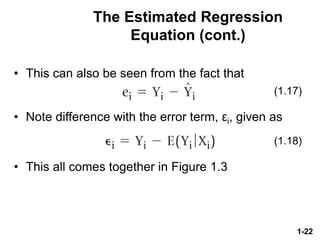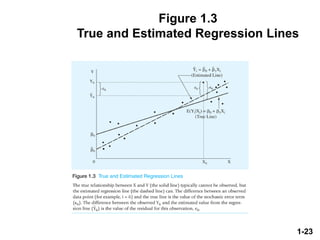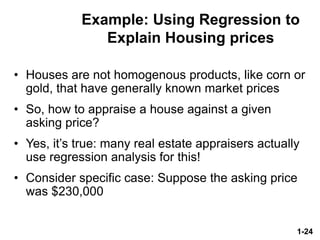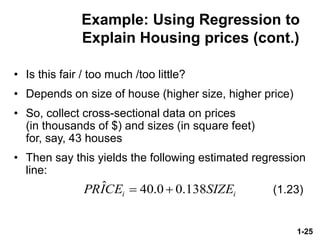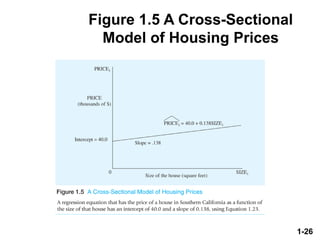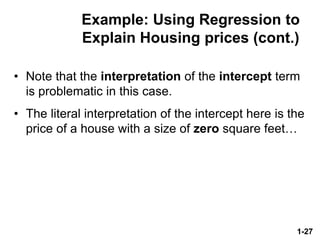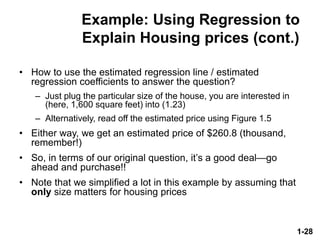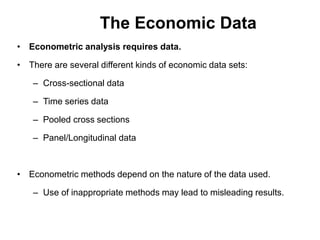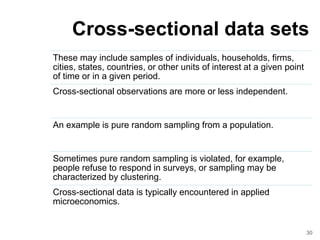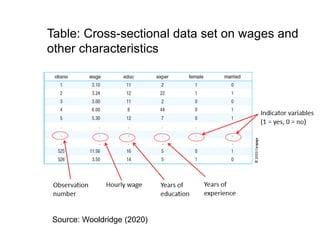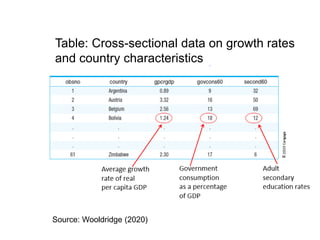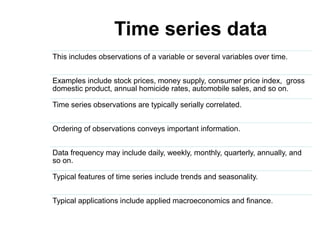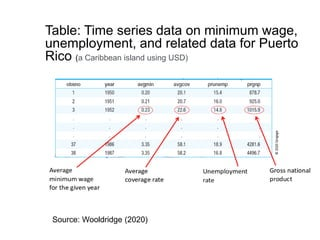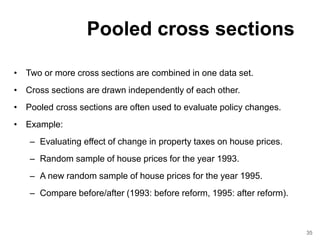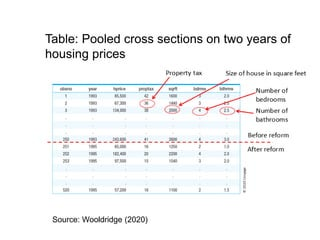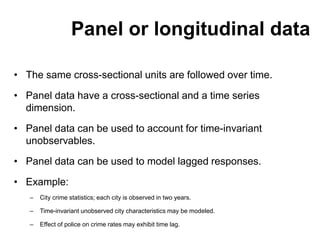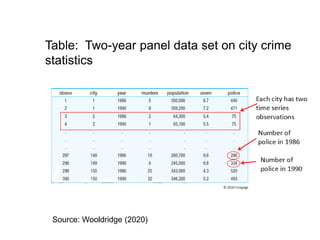1 von 39
Anzeige

### WEEK 1 Introduction.pdf

1. EIA2006 Basic Econometrics/ EIA2013 Econometrics 1
2. 1-1 What is Econometrics? • Econometrics literally means “economic measurement” • It is the quantitative measurement and analysis of actual economic and business phenomena— and so involves: – economic theory – Statistics – Math – observation/data collection
3. 1-2 What is Econometrics? (cont.) • Three major uses of econometrics: – Describing economic reality – Testing hypotheses about economic theory – Forecasting future economic activity • So econometrics is all about questions: the researcher (YOU!) first asks questions and then uses econometrics to answer them
4. Economic Theory Econometric Model Data Estimation Specification testing & diagnostic checking Is the model adequate? If Yes Tests of any hypotheses Using the model for predictions & policy If No Steps involved in an econometric analysis of economic models
5. 1-5 Example • Consider the general and purely theoretical relationship: Q = f(P, Ps, Yd) (1.1) • Econometrics allows this general and purely theoretical relationship to become explicit: Q = 27.7 – 0.11P + 0.03Ps + 0.23Yd (1.2)
6. 1-6 What is Regression Analysis? • Economic theory can give us the direction of a change, e.g. the change in the demand for dvd’s following a price decrease (or price increase) • But what if we want to know not just “how?” but also “how much?” • Then we need: – A sample of data – A way to estimate such a relationship • one of the most frequently ones used is regression analysis
7. 1-7 What is Regression Analysis? (cont.) • Formally, regression analysis is a statistical technique that attempts to “explain” movements in one variable, the dependent variable, as a function of movements in a set of other variables, the independent (or explanatory) variables, through the quantification of a single equation
8. 1-8 Example • Return to the example from before: Q = f(P, Ps, Yd) (1.1) • Here, Q is the dependent variable and P, Ps, Yd are the independent variables • Don’t be deceived by the words dependent and independent, however – A statistically significant regression result does not necessarily imply causality – We also need: • Economic theory • Common sense
9. 1-9 Single-Equation Linear Models • The simplest example is: Y = β0 + β1X (1.3) • The βs are denoted “coefficients” – β0 is the “constant” or “intercept” term – β1 is the “slope coefficient”: the amount that Y will change when X increases by one unit; for a linear model, β1 is constant over the entire function
10. 1-10 Figure 1.1 Graphical Representation of the Coefficients of the Regression Line
11. 1-11 Single-Equation Linear Models (cont.) • Application of linear regression techniques requires that the equation be linear—such as (1.3) • By contrast, the equation Y = β0 + β1X2 (1.4) is not linear • What to do? First define Z = X2 (1.5) • Substituting into (1.4) yields: Y = β0 + β1Z (1.6) • This redefined equation is now linear (in the coefficients β0 and β1 and in the variables Y and Z)
12. 1-12 Single-Equation Linear Models (cont.) • Is (1.3) a complete description of origins of variation in Y? • No, at least four sources of variation in Y other than the variation in the included Xs: • Other potentially important explanatory variables may be missing (e.g., X2 and X3) • Measurement error • Incorrect functional form • Purely random and totally unpredictable occurrences • Inclusion of a “stochastic error term” (ε) effectively “takes care” of all these other sources of variation in Y that are NOT captured by X, so that (1.3) becomes: Y = β0 + β1X + ε (1.7)
13. 1-13 Single-Equation Linear Models (cont.) • Two components in (1.7): – deterministic component (β0 + β1X) – stochastic/random component (ε) • Why “deterministic”? – Indicates the value of Y that is determined by a given value of X (which is assumed to be non-stochastic) – Alternatively, the det. comp. can be thought of as the expected value of Y given X—namely E(Y|X)—i.e. the mean (or average) value of the Ys associated with a particular value of X – This is also denoted the conditional expectation (that is, expectation of Y conditional on X)
14. 1-14 Example: Aggregate Consumption Function • Aggregate consumption as a function of aggregate income may be lower (or higher) than it would otherwise have been due to: – consumer uncertainty—hard (impossible?) to measure, i.e. is an omitted variable – Observed consumption may be different from actual consumption due to measurement error – The “true” consumption function may be nonlinear but a linear one is estimated (see Figure 1.2 for a graphical illustration) – Human behavior always contains some element(s) of pure chance; unpredictable, i.e. random events may increase or decrease consumption at any given time • Whenever one or more of these factors are at play, the observed Y will differ from the Y predicted from the deterministic part, β0 + β1X
15. 1-15 Figure 1.2 Errors Caused by Using a Linear Functional Form to Model a Nonlinear Relationship
16. 1-16 Extending the Notation • Include reference to the number of observations – Single-equation linear case: Yi = β0 + β1Xi + εi (i = 1,2,…,N) (1.10) • So there are really N equations, one for each observation • the coefficients, β0 and β1, are the same • the values of Y, X, and ε differ across observations
17. 1-17 Extending the Notation (cont.) • The general case: multivariate regression Yi = β0 + β1X1i + β2X2i + β3X3i + εi (i = 1,2,…,N) (1.11) • Each of the slope coefficients gives the impact of a one-unit increase in the corresponding X variable on Y, holding the other included independent variables constant (i.e., ceteris paribus) • As an (implicit) consequence of this, the impact of variables that are not included in the regression are not held constant.
18. 1-18 Example: Wage Regression • Let wages (WAGE) depend on: – years of work experience (EXP) – years of education (EDU) – gender of the worker (GEND: 1 if male, 0 if female) • Substituting into equation (1.11) yields: WAGEi = β0 + β1EXPi + β2EDUi + β3GENDi + εi (1.12)
19. 1-19 Indexing Conventions • Subscript “i” for data on individuals (so called “cross section” data) • Subscript “t” for time series data (e.g., series of years, months, or days—daily exchange rates, for example ) • Subscript “it” when we have both (for example, “panel data”)
20. 1-20 The Estimated Regression Equation • The regression equation considered so far is the “true”—but unknown—theoretical regression equation • Instead of “true,” might think about this as the population regression vs. the sample/estimated regression • How do we obtain the empirical counterpart of the theoretical regression model (1.10)? • It has to be estimated • The empirical counterpart to (1.10) is: (1.16) • The signs on top of the estimates are denoted “hat,” so that we have “Y-hat,” for example i i X Y 1 0 ˆ ˆ ˆ   + =
21. 1-21 The Estimated Regression Equation (cont.) • For each sample we get a different set of estimated regression coefficients • Y is the estimated value of Yi (i.e. the dependent variable for observation i); similarly it is the prediction of E(Yi|Xi) from the regression equation • The closer Y is to the observed value of Yi, the better is the “fit” of the equation • Similarly, the smaller is the estimated error term, ei, often denoted the “residual,” the better is the fit
22. 1-22 The Estimated Regression Equation (cont.) • This can also be seen from the fact that (1.17) • Note difference with the error term, εi, given as (1.18) • This all comes together in Figure 1.3
23. 1-23 Figure 1.3 True and Estimated Regression Lines
24. 1-24 Example: Using Regression to Explain Housing prices • Houses are not homogenous products, like corn or gold, that have generally known market prices • So, how to appraise a house against a given asking price? • Yes, it’s true: many real estate appraisers actually use regression analysis for this! • Consider specific case: Suppose the asking price was \$230,000
25. 1-25 Example: Using Regression to Explain Housing prices (cont.) • Is this fair / too much /too little? • Depends on size of house (higher size, higher price) • So, collect cross-sectional data on prices (in thousands of \$) and sizes (in square feet) for, say, 43 houses • Then say this yields the following estimated regression line: (1.23) i i SIZE CE I PR 138 . 0 0 . 40 ˆ + =
26. 1-26 Figure 1.5 A Cross-Sectional Model of Housing Prices
27. 1-27 Example: Using Regression to Explain Housing prices (cont.) • Note that the interpretation of the intercept term is problematic in this case. • The literal interpretation of the intercept here is the price of a house with a size of zero square feet…
28. 1-28 Example: Using Regression to Explain Housing prices (cont.) • How to use the estimated regression line / estimated regression coefficients to answer the question? – Just plug the particular size of the house, you are interested in (here, 1,600 square feet) into (1.23) – Alternatively, read off the estimated price using Figure 1.5 • Either way, we get an estimated price of \$260.8 (thousand, remember!) • So, in terms of our original question, it’s a good deal—go ahead and purchase!! • Note that we simplified a lot in this example by assuming that only size matters for housing prices
29. • Econometric analysis requires data. • There are several different kinds of economic data sets: – Cross-sectional data – Time series data – Pooled cross sections – Panel/Longitudinal data • Econometric methods depend on the nature of the data used. – Use of inappropriate methods may lead to misleading results. The Economic Data
30. Cross-sectional data sets These may include samples of individuals, households, firms, cities, states, countries, or other units of interest at a given point of time or in a given period. Cross-sectional observations are more or less independent. An example is pure random sampling from a population. Sometimes pure random sampling is violated, for example, people refuse to respond in surveys, or sampling may be characterized by clustering. Cross-sectional data is typically encountered in applied microeconomics. 30
31. obsno wage educ exper female married 1 3.10 11 2 1 0 2 3.24 12 22 1 1 3 3.00 11 2 0 0 4 6.00 8 44 0 1 5 5.30 12 7 0 1 . . . . . . . . . . . . . . . . . . 525 11.56 16 5 0 1 526 3.50 14 5 1 0 Table: Cross-sectional data set on wages and other characteristics Source: Wooldridge (2020)
32. obsno country gpcrgdp govcons60 second60 1 Argentina 0.89 9 32 2 Austria 3.32 16 50 3 Belgium 2.56 13 69 4 Bolivia 1.24 18 12 . . . . . . . . . . . . . . . 61 Zimbabwe 2.30 17 6 Table: Cross-sectional data on growth rates and country characteristics Source: Wooldridge (2020)
33. Time series data This includes observations of a variable or several variables over time. Examples include stock prices, money supply, consumer price index, gross domestic product, annual homicide rates, automobile sales, and so on. Time series observations are typically serially correlated. Ordering of observations conveys important information. Data frequency may include daily, weekly, monthly, quarterly, annually, and so on. Typical features of time series include trends and seasonality. Typical applications include applied macroeconomics and finance.
34. obsno year avgmin avgcov prunemp prgnp 1 1950 0.20 20.1 15.4 878.7 2 1951 0.21 20.7 16.0 925.0 3 1952 0.23 22.6 14.8 1015.9 . . . . . . . . . . . . . . . . . . 37 1986 3.35 58.1 18.9 4281.6 38 1987 3.35 58.2 16.8 4496.7 Table: Time series data on minimum wage, unemployment, and related data for Puerto Rico (a Caribbean island using USD) Source: Wooldridge (2020)
35. Pooled cross sections • Two or more cross sections are combined in one data set. • Cross sections are drawn independently of each other. • Pooled cross sections are often used to evaluate policy changes. • Example: – Evaluating effect of change in property taxes on house prices. – Random sample of house prices for the year 1993. – A new random sample of house prices for the year 1995. – Compare before/after (1993: before reform, 1995: after reform). 35
36. obsno year hprice proptax sqrft bdrms bthrms 1 1993 85,500 42 1600 3 2 2 1993 67,300 36 1440 3 2 3 1993 134,000 38 2000 4 2 . . . . . . . . . . . . . . . . . . . . . 250 1993 243,600 41 2600 4 3 251 1995 65,000 16 1250 2 1 252 1995 182,400 20 2200 4 2 253 1995 97,500 15 1540 3 2 . . . . . . . . . . . . . . . . . . . . . 520 1995 57,200 16 1100 2 1 Table: Pooled cross sections on two years of housing prices Source: Wooldridge (2020)
37. Panel or longitudinal data • The same cross-sectional units are followed over time. • Panel data have a cross-sectional and a time series dimension. • Panel data can be used to account for time-invariant unobservables. • Panel data can be used to model lagged responses. • Example: – City crime statistics; each city is observed in two years. – Time-invariant unobserved city characteristics may be modeled. – Effect of police on crime rates may exhibit time lag.
38. obsno city year murders population unem police 1 1 1986 5 350,000 8.7 440 2 1 1990 8 359,200 7.2 471 3 2 1986 2 64,300 5.4 75 4 2 1990 1 65,100 5.5 75 . . . . . . . . . . . . . . . . . . . . . 297 149 1986 10 260,700 9.6 286 298 149 1990 6 245,000 9.8 334 299 150 1986 25 543,000 4.3 520 300 150 1990 32 546,200 5.2 493 Table: Two-year panel data set on city crime statistics Source: Wooldridge (2020)
Anzeige